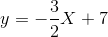# High School Math : How to find the equation of a parallel line

## Example Questions

### Example Question #61 : Algebra I

What line is parallel to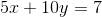through the point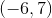?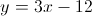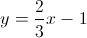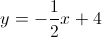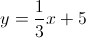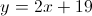Explanation:

The given line can be rewritten as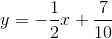, which has slope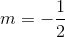.

If the new line is parallel to the old line, it must have the same slope.  So we use the point-slope form of an equation to calculate the new intercept.becomes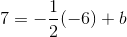where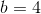.

So the equation of the parallel line is.

### Example Question #1 : How To Find The Equation Of A Parallel Line

Find the equation of a line parallel to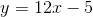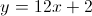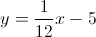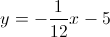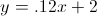Explanation:

Since parallel lines share the same slope, the only answer that works is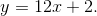### Example Question #3 : How To Find The Equation Of A Parallel Line

Given the equation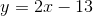and the point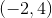, find a line through the point that is parallel to the given line.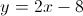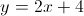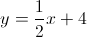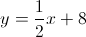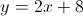Explanation:

In order for two lines to be parallel, they must have the same slope. The slope of the given line is, so we know that the line going through the given point also has to have a slope of. Using the point-slope formula,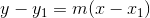,

whererepresents the slope and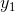and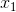represent the given points, plug in the points given and simplify into standard form: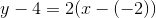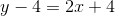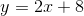### Example Question #4 : How To Find The Equation Of A Parallel Line

What line is parallel to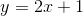through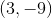?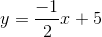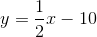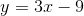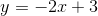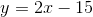Explanation:

Parallel lines have the same slopes.  The slope for the given equation is.  We can use the slope and the new point in the slope intercept equation to solve for the intercept: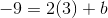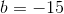Therefore the new equation becomes:### Example Question #5 : How To Find The Equation Of A Parallel Line

What line is parallel to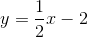through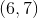?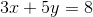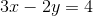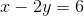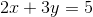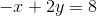Explanation:

Parallel lines have the same slope.  The slope of the given line is.

Find the line with slopethrough the pointby plugging this informatuon into the slope intercept equation,: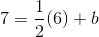, which gives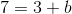.

Solve forby subtractingfrom both sides to get.

Then the parallel line equation becomes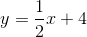, and converting to standard form gives.

### Example Question #1 : How To Find The Equation Of A Parallel Line

Find the equation of a line parallel to the line that goes through points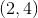and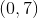.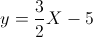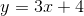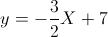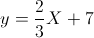Parallel lines share the same slope. Because the slope of the original line is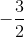, the correct answer must have that slope, so the correct answer is